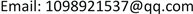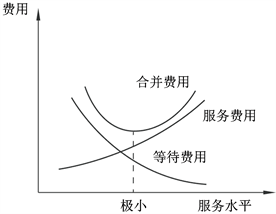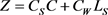﻿ 基于排队论的校园快递高峰服务系统优化研究 Optimization Analysis of Campus Express Peak Service System Based on Queuing Theory

Management Science and Engineering
Vol. 07  No. 04 ( 2018 ), Article ID: 27874 , 8 pages
10.12677/MSE.2018.74033

Optimization Analysis of Campus Express Peak Service System Based on Queuing Theory

Nan Gao

Beijing Jiaotong University, BeijingReceived: Nov. 15th, 2018; accepted: Nov. 29th, 2018; published: Dec. 6th, 2018ABSTRACT

In order to solve the problems faced in the campus express peak service system, this paper first uses the knowledge of queuing theory to make the service system reach the steady state as the premise, and minimize the total cost of the system as the goal to construct the optimization model. Secondly, the paper collects data from an express delivery agency around Xueyuan Apartment in Beijing Jiaotong University, and calculates the main indicators. Finally, the optimization results of the campus express peak service system are given by the optimization model, which can provide reference for the decision-making of the campus express peak service system.

Keywords:Campus Express, Queuing Theory, Optimization ResearchCopyright © 2018 by author and Hans Publishers Inc.1. 引言

21世纪随着互联网、物流业的快速发展  ，带动了网购潮流的兴起与热衷。网上购物已被越来越多的消费者接受，大学生作为一类消费综合性很强，又是走在潮流前沿的庞大群体，这种新潮且快捷的购物方式在大学生中很受青睐。大学生成为当代网购的一支主力军，因而对校园快递网点的多样化需求也越来越多、越来越细。学生的网购频率很高，特别是像“女王节”、“双十一”、“双十二”这种促销日，大量的网购使得快递网点货物堆积如山，这已经是校园快递的常态了，所以校园快递网点在高峰期提高派件服务效率尤为重要。校园快递高峰期是快递点问题高发的一个时段，“校园快递”在高峰期变成“校园慢递”，派件现场出现一系列问题，会影响到客户对其的满意度  。在学校，快递员不会直接将快递送到学生宿舍门口，而是需要我们到代收点领取。由于快递代收点空间和服务人员有限，而大学生领取快递的时间又相对集中，所以势必会出现排队等待的现象。那么对于快递代收点，究竟设置几位服务人员，既能不浪费快递代收点成本，又不会让顾客长时间等待，此类问题随之出现。本文运用排队论的知识对校园快递高峰期取件问题进行优化，旨在实现快递网点成本最小化，提高取件速度和顾客满意度。

2. 校园快递代收点问题描述

2.1. 校园快递代收点基本情况

2.2. 高峰期取件存在的问题

2.2.1. 高峰期取件时间长

2.2.2. 闲期人力资源浪费

3. 高峰期服务系统优化模型的建立

3.1. 排队论标准的M/M/C模型

1) 服务强度

$\rho =\frac{\lambda }{cu}$ (3-1)

2) 系统中没有顾客的概率

${p}_{0}=\frac{1}{\underset{n=0}{\overset{c-1}{\sum }}\left[{\left(\lambda /u\right)}^{n}/n!\right]+\left[{\left(\lambda /u\right)}^{c}/c!\right]\left[c\mu /\left(c\mu -\lambda \right)\right]}$ (3-2)

3) 系统中平均排队长

${L}_{q}=\frac{{\left(c\rho \right)}^{c}\rho }{c!{\left(1-\rho \right)}^{2}}{p}_{0}$ (3-3)

4) 系统中平均队列长

${L}_{S}={L}_{q}+\frac{\lambda }{u}$ (3-4)

5) 顾客在系统中的平均逗留时间

${W}_{S}=\frac{{L}_{S}}{\lambda }$ (3-5)

6) 顾客在系统中的平均等待时间

${W}_{q}=\frac{{L}_{q}}{\lambda }=\frac{{\left(\lambda /\mu \right)}^{c}\mu }{\left(c-1\right)!{\left(c\mu -\lambda \right)}^{2}}{P}_{0}$ (3-6)

3.2. 排队论最优化模型Figure 1. Queuing system costs

M/M/C模型在稳定状态下，单位时间总成本(服务费用与等待费用相加)的目标值为(3-7)

 (3-8)

$\left\{\begin{array}{l}{C}_{S}{C}^{*}+{C}_{W}L\left({C}^{*}\right)\le {C}_{S}\left({C}^{*}-1\right)+{C}_{W}L\left({C}^{*}-1\right)\\ {C}_{S}{C}^{*}+{C}_{W}L\left({C}^{*}\right)\le {C}_{S}\left({C}^{*}+1\right)+{C}_{W}L\left({C}^{*}+1\right)\end{array}$ (3-9)

$L\left({C}^{*}\right)-L\left({C}^{*}+1\right)\le \frac{{C}_{S}}{{C}_{W}}\le L\left({C}^{*}-1\right)-L\left({C}^{*}\right)$ (3-10)

$C=1,2,3,\cdots$ 时计算LS的值，求LS相邻两个的差。因为  已求得，要得到最小的 ${C}^{*}$ ，需要观察 $\frac{{C}_{S}}{{C}_{W}}$

4. 算例分析

4.1. 平均达到率

(数据资料来源：通过本人调研和整理所得)。

1) 平均服务率μTable 3. Service time statistics during peak hours

(数据资料来源：通过本人调研和整理所得)。

$\rho =\frac{\lambda }{c\mu }=\frac{150}{2×67}=1.12>1$ ，此状态下高峰期队列会无限制延长下去，不能使系统到达平衡。排队等待的

2) 顾客最大等待时间

4.2. 相关指标的计算Table 4. Open various operating indicators under different windows

4.3. 基于成本优化的服务窗口数的确定

$L\left({C}^{*}\right)-L\left({C}^{*}+1\right)\le \frac{{C}_{S}}{{C}_{W}}\le L\left({C}^{*}-1\right)-L\left(C*\right)$Table 5. Number of service personnel based on cost optimization and total cost

5. 结论

Optimization Analysis of Campus Express Peak Service System Based on Queuing Theory[J]. 管理科学与工程, 2018, 07(04): 272-279. https://doi.org/10.12677/MSE.2018.74033

1. 1. 孙水波, 韩淑英. 现代信息技术在物流管理中的应用[J]. 物流科技, 2008(10): 44-48.

2. 2. 刘珍, 慕艳平. 爆仓时代校园物流如何应对[J]. 中国电子商务, 2013(5): 29-30.

3. 3. 梁剑, 程文明, 张亚. 铁路集装箱中心站大门系统优化设计[J]. 中国铁路, 2008(12): 6-8.

4. 4. 周薇, 罗荣桂, 田磊. 排队论在计算机存储系统性能中的应用和分析[J]. 微计算机信息, 2006, 22(21): 271-272.

5. 5. 吴耀华, 王胜力, 王常香. 排队论在物流规划中的应用[J]. 物流科技, 2004(5): 53-58.

6. 6. Li, C.-P. and Neely, M.J. (2014) Solving Convex Optimization with Side Constraints in a Multi-Class Queue by Adaptive cμ Rule. Queueing Systems, 77, 331-372.
https://doi.org/10.1007/s11134-013-9377-3

7. 7. Danaher, A.R. (2000) Transit Capacity and Quality of Service Manual. Transportation Research Board, Washington DC.

8. 8. 胡运权. 运筹学基础及应用[M]. 第5版. 北京: 高等教育出版社, 2008: 312-313.

9. 9. 马超群, 兰秋军, 周忠宝. 运筹学[M]. 长沙: 湖南大学出版社, 2010: 251-252.

10. 10. 韩伯棠. 管理运筹学[M]. 第3版. 北京: 高等教育出版社, 2010: 384-385.

11. 11. 古贞, 单晓曦, 邹亚清, 等. 大学校园设立快递超市的可行性分析研究——以南京高校为例[J]. 物流工程与管理, 2014, 36(5): 206-207.

12. 12. de Lange, R., Samoilovich, I. and van der Rhee, B. (2013) Virtual Queuing at Airport Security Lanes. European Journal of Operational Research, 225, 153-165.
https://doi.org/10.1016/j.ejor.2012.09.025